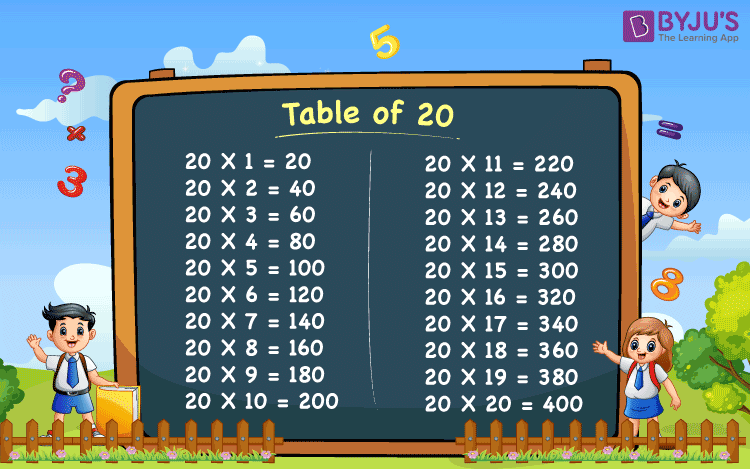# Table of 20

Table of 20 is essential for both school going students and for students who are preparing for competitive exams, other national and international exams. Memorizing the table of 20 will help the students to solve complicated mathematical problems faster. To help students learn the table of 20 we have provided 20 times table here.

## Table of 20 Chart## Multiplication Table of 20

 20 X 1 = 20 20 X 2 = 40 20 X 3 = 60 20 X 4 = 80 20 X 5 = 100 20 X 6 = 120 20 X 7 = 140 20 X 8 = 160 20 X 9 = 180 20 X 10 = 200 20 X 11 = 220 20 X 12 = 240 20 X 13 = 260 20 X 14 = 280 20 X 15 = 300 20 X 16 = 320 20 X 17 = 340 20 X 18 = 360 20 X 19 = 380 20 X 20 = 400

Visit BYJU’S for latest CBSE syllabus. We also provide CBSE notes for each and every class.

Get More Maths Tables:-

## Frequently Asked Questions on Table Of 20

Q1

### What is the multiplication table of 20?

The multiplication table of 20 gives the multiples of 20. The table of 20 is obtained by multiplying 20 with the different natural numbers.

Q2

### What are the first 5 multiples of 20?

The first 5 multiples of 20 are 20, 40, 60, 80 and 100.

Q3

### Write the table of 20 up to the first 10 natural numbers?

The table of 20 upto the first 10 times are:
20 × 1 = 20
20 × 2 = 40
20 × 3 = 60
20 × 4 = 80
20 × 5 = 100
20 × 6 = 120
20 × 7 = 140
20 × 8 = 160
20 × 9 = 180
20 × 10 = 200.

Q4

### Find the product of 10 and 20?

The product of 10 and 20 is 200.
I.e., 20 × 10 = 200.

Q5

### What number should be multiplied with 20 to get 140?

7 should be multiplied with 20 to get 140.
I.e., 7 × 20 = 140

Q6

### Find the value of a is 20a = 400.

20a = 400
Hence, a = 400/20 = 20
Therefore, the value of a is 20.

Q7

### What is the smallest multiple of 20?

We know that the smallest multiple of a number is the number itself.
Hence the smallest multiple of 20 is 20.

Q8

### What is the fifth multiple of 20?

The fifth multiple of 20 is 100.
I.e, 20 × 5 = 100.

Test your knowledge on Table Of 20